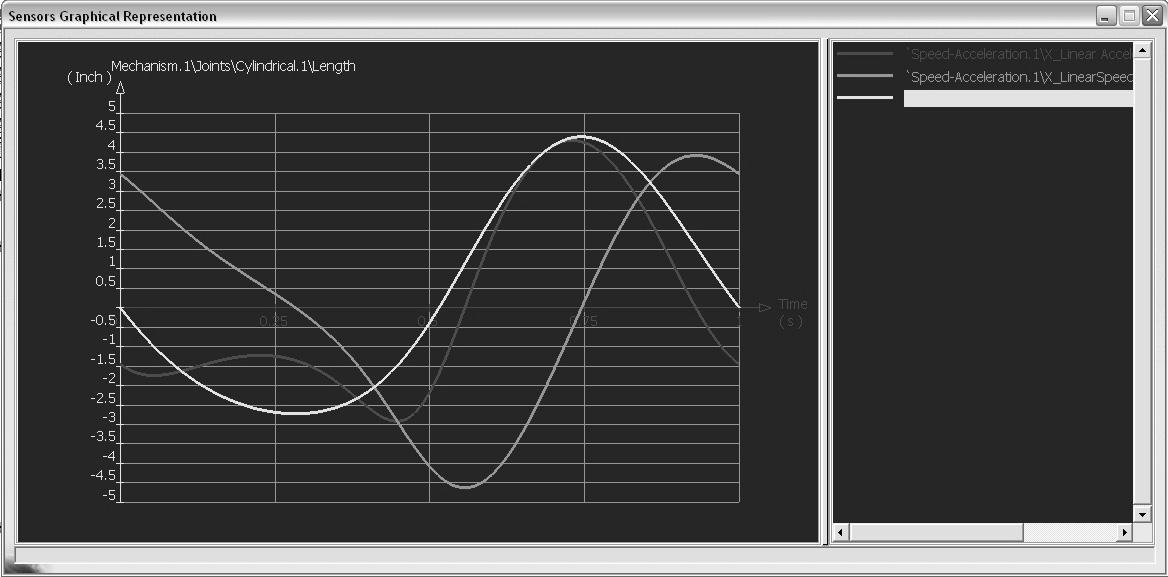# Tutorial : Using Laws in the motion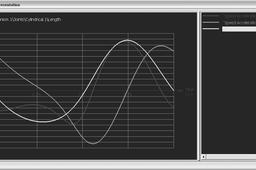The tutorial continues here.

1. ### Step 1:

Note that the Speed and Acceleration.1 has appeared in the tree.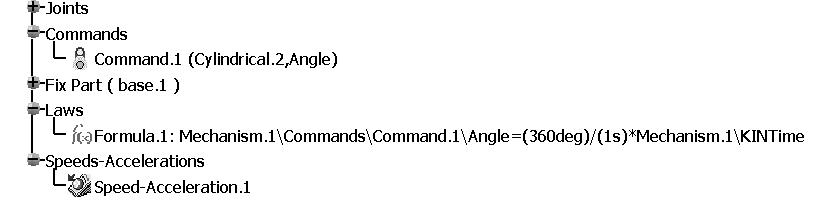2. ### Step 2:

Having entered the required kinematic relation and designated the vertex on the block as the point to collect data on, we will simulate the mechanism. Click on Simulation with Laws icon in the Simulation toolbar .
This results in the Kinematics Simulation pop up box shown below.
Note that the default time duration is 10 seconds.
To change this value, click on the button. In the resulting pop up box,
change the time duration to 1s. This is the time duration for the crank to make one full revolution.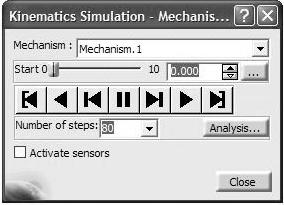3. ### Step 3:

The scroll bar now moves up to 1s.
Check the Activate sensors box, at the bottom left corner.
You will next have to make certain selections from the accompanying Sensors box.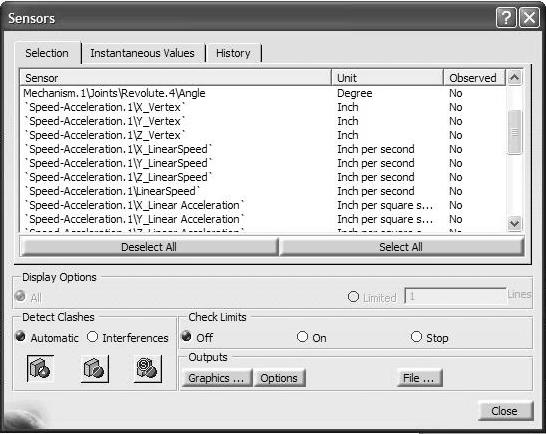4. ### Step 4:

Observing that the coordinate direction of interest is X, click on the following items to record position, velocity, and acceleration of the block:

Mechanism.1\Joints\Cylindrical.1\Length
Speed-Acceleration.1\X_LinearSpeed
Speed-Acceleration.1\X_LinearAcceleration

As you make selections in this window, the last column in the Sensors box, changes to Yes for the corresponding items. This is shown on the next page. Do not close the Sensors box after you have made your selection (leave it open to generate results).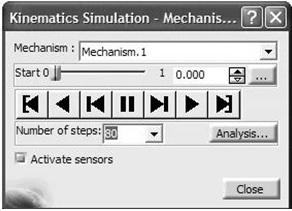5. ### Step 5:

Also, change the Number of steps to 80.
The larger this number, the smoother the
velocity and acceleration plots will be.6. ### Step 6:

Once the crank reaches the end, click on the Graphics button in the
Sensor box. The result is the plot of the position, velocity and acceleration all on the same axis (but with the vertical axis units corresponding to whichever one of the three outputs is highlighted in the right side of the window). Click on each of the three outputs to see the corresponding axis units for each output. The three plots for position (corresponding to cylindrical joint Length), velocity (X_LinearSpeed), and acceleration (X_Linear_Acceleration) are shown below.